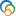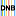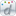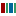The Library of Congress > Linked Data Service > LC Subject Headings (LCSH)

# Yang-Baxter equation

• ### Variants

• Baxter-Yang equation
• Factorization equation
• Star-triangle relation
• Triangle equation

• ### Closely Matching Concepts from Other Schemes

•Yang-Baxter equation•Yang-Baxter-Gleichung•Yang-Baxter, Équation de•Yang–Baxter equationLabel from public data source Wikidata
• ### Sources

• found: Work cat.: Yang-Baxter equation in integrable systems, 1990:Preface (also known as: Star-triangle relation; Triangle equation; factorization equation)
• notfound: NASA;INIS:Thes.;INSPEC;Random House;McGraw-Hill dict. sci. tech.;Encyc. phys. sci. tech.;Encyc. physics
• ### LC Classification

• QC174.52.Y36

• ### Change Notes

• 1989-12-22: new
• 1990-02-06: revised